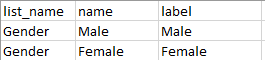# Piping custom text

Hello Dears,

Kindly is there a way to piping a custom text as below:
Q1 Gender [Male/Female]

Q2. {'xx"} we are happy to …

we need

• if the answer of Q1 is Male so piping {'xx"} as Sir.
• if the answer of Q1 is Female so piping {'xx"} as Madam.

Hi @surveyhub,

1. You can create a `calculate` question with an IF statement:

if( \${Q1} = ‘Male’, “Sir”, if( \${Q1} = ‘Female’, “Madam”, “”))

Note that, I used 2 IF statements for getting an empty value if nothing is selected. You can alternatively solve the issue with 1 IF statement.

1. Then start your Q2 with:

\${calculation} we are happy to,

This is the screenshot of the survey sheet of IF statement in XLS:

This is the choices sheet:2 Likes

Thanks a lot, it really works

1 Like